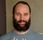cancel
Showing results for
Did you mean:

Fabric is Generally Available. Browse Fabric Presentations. Work towards your Fabric certification with the Cloud Skills Challenge.Resolver I

## Calculate Month/Quarter base on Year and week Number

Hi,

I need some advice about to create two columns, I am wondering if this could be a Measure or calculated column, here is some explanation about the topic:

I have two columns in my query, YEAR and WEEK number, Do I need is two create two new column to show:

1.  Months, using Year and Week column as source value to create a new column with the months, eg:

YEAR= 2019/WEEK = 1 == MONTH = JANUARY

2. Quarter, using Year and Week columns as source values to create a new column with the Quarters, eg:

YEAR= 2019/WEEK = 1 == QUARTER= 1

1 ACCEPTED SOLUTIONResident Rockstar

Hi @Rigolleto ,

you should do this by using calculated columns, not measures. It is possible to do this in Power Query, here is how to do it with DAX.

First create column with the date of the start of the week:

``````WeekStartDate =
var _first7daysOfYear = GENERATESERIES(date('Table'[year];1;1);DATE('Table'[year];1;7))
var _firstMondayDate = SUMMARIZE(filter(_first7Weekdays;[weekday]=1);[Value])
var _firstMondayWeek = SUMMARIZE(filter(_first7Weekdays;[weekday]=1);[weeknum])
return
SWITCH(
true();
_firstMondayWeek=2 && 'Table'[week]>=2;_firstMondayDate+(7*('Table'[week]-2));
_firstMondayWeek=2 && 'Table'[week]=1;DATE(2019;1;1);
_firstMondayWeek=1;_firstMondayDate+(7*('Table'[week]-1));
BLANK())``````

This code sets the start date of week 1 to 01.01.2019. For the other weeks it sets the start date to the mondat of that week.

Then use this to find the month:

``Month = STARTOFMONTH('Table'[WeekStartDate])``

and quater

``Quater = "Q" & FORMAT(STARTOFQUARTER('Table'[WeekStartDate]); "Q-yyyy")``

Keep in mind that this code does not take into account that some years will start with week 53. Also, as most month changes occurs within a week, in this code only the start of the week is used to determine if the week belongs in a month or quater.

Cheers,
Sturla

If this post helps, then please consider Accepting it as the solution. Kudos are nice too.Resident Rockstar

Hi @Rigolleto ,

you should do this by using calculated columns, not measures. It is possible to do this in Power Query, here is how to do it with DAX.

First create column with the date of the start of the week:

``````WeekStartDate =
var _first7daysOfYear = GENERATESERIES(date('Table'[year];1;1);DATE('Table'[year];1;7))
var _firstMondayDate = SUMMARIZE(filter(_first7Weekdays;[weekday]=1);[Value])
var _firstMondayWeek = SUMMARIZE(filter(_first7Weekdays;[weekday]=1);[weeknum])
return
SWITCH(
true();
_firstMondayWeek=2 && 'Table'[week]>=2;_firstMondayDate+(7*('Table'[week]-2));
_firstMondayWeek=2 && 'Table'[week]=1;DATE(2019;1;1);
_firstMondayWeek=1;_firstMondayDate+(7*('Table'[week]-1));
BLANK())``````

This code sets the start date of week 1 to 01.01.2019. For the other weeks it sets the start date to the mondat of that week.

Then use this to find the month:

``Month = STARTOFMONTH('Table'[WeekStartDate])``

and quater

``Quater = "Q" & FORMAT(STARTOFQUARTER('Table'[WeekStartDate]); "Q-yyyy")``

Keep in mind that this code does not take into account that some years will start with week 53. Also, as most month changes occurs within a week, in this code only the start of the week is used to determine if the week belongs in a month or quater.

Cheers,
Sturla

If this post helps, then please consider Accepting it as the solution. Kudos are nice too.Announcements#### Power BI Monthly Update - November 2023

Check out the November 2023 Power BI update to learn about new features.#### The largest Power BI and Fabric virtual conference

130+ sessions, 130+ speakers, Product managers, MVPs, and experts. All about Power BI and Fabric. Attend online or watch the recordings.Top Solution Authors
Top Kudoed Authors
Users online (6,215)